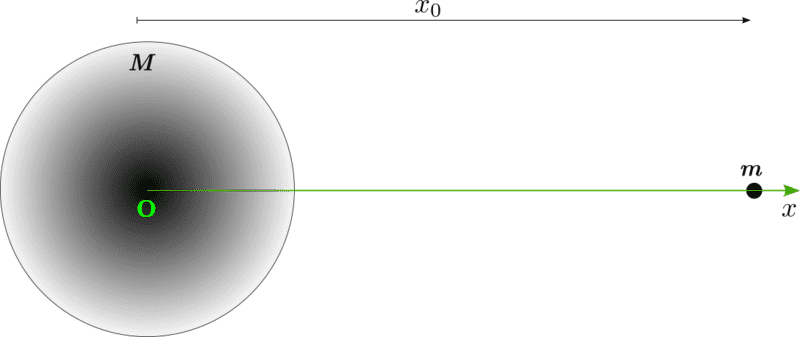# The gravitating of a small mass towards a big mass

• brotherbobby

#### brotherbobby

Homework Statement
A large mass of ##10^8## kg is held in free space at a certain point. A small mass of 1 kg exists at a point 1000 km (##10^6\;\text{m}##) away from the larger mass at the start of motion. Answer the following questions :
(1) Write the velocity of the smaller mass as function(s) of its space coordinate and time, assuming the location of the larger mass to be at the origin, the motion taking place along a line and the start of motion to be at ##t=0##.
(2) What is the location of the smaller mass at a given time ##t##?
(3) When will the smaller mass collide with the bigger mass?
(4) With what velocity will the bigger mass collide with the smaller mass?
Relevant Equations
From Newton's gravitation formula, the acceleration due to gravity ##g=\frac{d^2x}{dt^2}= \frac{GM}{x^2}##, where ##x## is the distance of separation between the masses ##M## and ##m## at a given instant.Diagram :
I draw a picture of the problem situation and paste it to the right.

Attempt : Let me assume that the position of the smaller mass ##m## at a given instant of time ##t## is ##x(t)##.

(1) Gravitational potential energy ##\frac{GMm}{x} = \frac{1}{2}mv^2(x)##, where ##v(x)## is the velocity of the mass at that position. This simplifies to ##\boxed{v(x) = \sqrt{\frac{2GM}{x}}}##.
However, I do not know how to find the velocity as a function of the time ##t##, or ##v(t)##.
More crucially, I do not know how to solve the differential equation given in the Relevant Equations above : ##\frac{d^2x}{dt^2}= \frac{GM}{x^2}##. If I could, it would yield ##\frac{dx}{dt} = v(t)## and ##x(t)##, thereby answering questions 1 and 2 above.
Solving the differential equation would also lead to finding when will the masses collide and with what velocity, which would answers questions (3) and (4) above.

Request : A help or hint would be very welcome.

For solving the differential equation, note that you have $$v(x) = \sqrt{\frac{2GM}{x}}=\frac{dx}{dt}.$$ Can you separate variables and integrate?

I am bothered by the fact that the radius of the larger sphere is not given. How will one know where the smaller mass is when it collides? Check your source to see if you missed it. If the radius is not given, assume that it is ##R## and proceed.

On edit
Actually it should be $$\frac{dx}{dt} = -\sqrt{\frac{2GM}{x}}$$ because the distance ##x## decreases as time increases.

Last edited:
(1) Gravitational potential energy ##\frac{GMm}{x} = \frac{1}{2}mv^2(x)##, where ##v(x)## is the velocity of the mass at that position.
GPE is not ##\frac{GMm}{x}## (you forgot something) and there is no law that says GPE equals KE (or -KE). What does it say?

•PeroK, malawi_glenn, erobz and 1 other person
Can you prove this?
If the object with mass ##m \ll M## is dropped at rest at the distance ##x_1## from the centre of the object with mass ##M##, then
## \dfrac{mv^2}{2} - \dfrac{GMm}{x} = -\dfrac{GMm}{x_1}## where ##x \leq x_1## and ##v## is the velocity at position ##x##.
Hint: work-energy theorem.